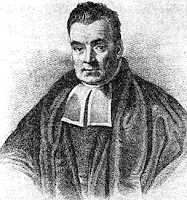# Evolution: A One-in-a-Billion ShotIt is no surprise that there are scientific problems with evolution. Its predictions are continually turning out to be false. It undoubtedly ranks number one in faulty expectations. For instance, one of its primary predictions, common descent, has badly failed. The reconciliation of the molecular and the visible, morphological, features has been a major problem in trying to resolve the evolutionary tree. The molecular and morphological features often indicate “strikingly different” evolutionary trees that cannot be explained as due to different methods being used.  Read more

## 7 Replies to “Evolution: A One-in-a-Billion Shot”

1. 1
Granville Sewell says:

Cornelius,

I think if you tried to classify forms of transportation, for each criterion used (type of fuel, number of wheels, medium in which they move, construction materials, for example), you would also get a very different evolutionary tree. This is because designers are not limited to using ideas from “closely related” forms.

Thanks for your posts, they are appreciated.

2. 2
Nakashima says:

Dr Hunter,

Your material might be improved by noting when you are passing on opinion that has been clearly marked as “Opinion” in the original. Presenting opinion as fact is something you have castigated others for doing, don’t do it yourself.

3. 3
anonym says:

Here’s an origin hypothesis. Just as Darwin’s idea of natural selection was to a large extent the worldview of classical economics (in particular Malthus) applied or extended to biology, was his conception of common descent – both the phylogenetic tree itself, and the comparative method as the means by which it is discovered – derived from nineteenth-century historical linguistics, another of the prestige ideas of the age? It seems that by the time he drew his first phylogenetic tree in 1837 Darwin was probably fairly well up to date with German philologists like Franz Bopp and Jacob Grimm, and according to David N. Stamos “the language analogy” was “his main analogy for species”. (See Chapter 3 of Darwin and the Nature of Species, to which I have partial access; I have no access to “Nationalism and the Reception of Jacob Grimm’s Deutsche Grammatik by English-Speaking Audiences” but it may be relevant too.)

If so, then there is the small irony that the tree of life is rooted, not very far below the base, in attempts to reconstruct the perfect language of the Garden of Eden. Two more-significant points remain relevant whatever the pedigree of the common descent idea. One is that since the early nineteenth century historical linguistics has turned out to be a particular lesson in the seductions and dangers of both “tree-thinking” and attempts to reconstruct origins using comparative methods.

4. 4
Prof_P.Olofsson says:

The calculations do not make any sense.

First of all, to compute the undonditional probability P(O), you need the conditional probabilities. Let’s say there are two theories, D(esign) and E(volution). Bayes’ formula states

P(E|O)=P(O|E)P(E)/P(O)

where

P(O)=P(O|E)P(E)+P(O|D)P(D)

The only probability we could even hypotheically hope to estimate is P(O|E). When you assume that P(O) is 1/2 and that P(O|E) is “small,” you are imposing conditions on the remaining probabilities.

Second, the probabilities are not “difficult” to gauge, they are impossible to gauge. What is P(D)? Some would say 0, some would say 1. Let’s not even get into the philosophical issues of assigning probabilities to events that have already happened…

Third, the values used are not “extremely conservative,” they are completely arbitrary.

5. 5
Prof_P.Olofsson says:

Let me be more specific. Hunter considers the ratio P(O|T)/P(O) which is the factor by which P(T) changes to P(T|O) after O has been observed. He claims that a ratio of 0.2 is “extremely conservative,” meaning that it is a very high value.

Let’s try some numbers. Following Hunter we want P(T) to be “very high” and P(O|T) to be “quite low.” Let’s take P(T)=0.999 and P(O|T)=0.1. What is the ratio P(O|T)/P(O)? We need P(O). By the law of total probability, assuming one competing hypothesis D:

P(O)=P(O|T)P(T)+P(O|D)P(D)

Since D is the complement of T, we have P(D)=0.001 which gives

P(O)=0.1*0.999+P(O|D)*0.001

and the ratio P(O|T)/P(O) becomes

0.1/(0.0999+P(O|D)*0.001)

Note that this ratio depends on P(O|D), the probability to observe O under the competing hypothesis. The largest possible value of P(O|D) is 1, in which case the ratio P(O|T)/P(O) equals

0.1/(0.0999+0.001)=0.99

Hence, the ratio P(O|T)/P(O) is at least 0.99, far above Hunter’s “extremely conservative” value of 0.2!

6. 6
Prof_P.Olofsson says:

PS. I would also like to point out the Cornelius is kind enough to let me discuss these issues on his blog, linked above.

7. 7
Heinrich says:

What is P(D)? Some would say 0, some would say 1.

Side-step it with Bayes Factors?